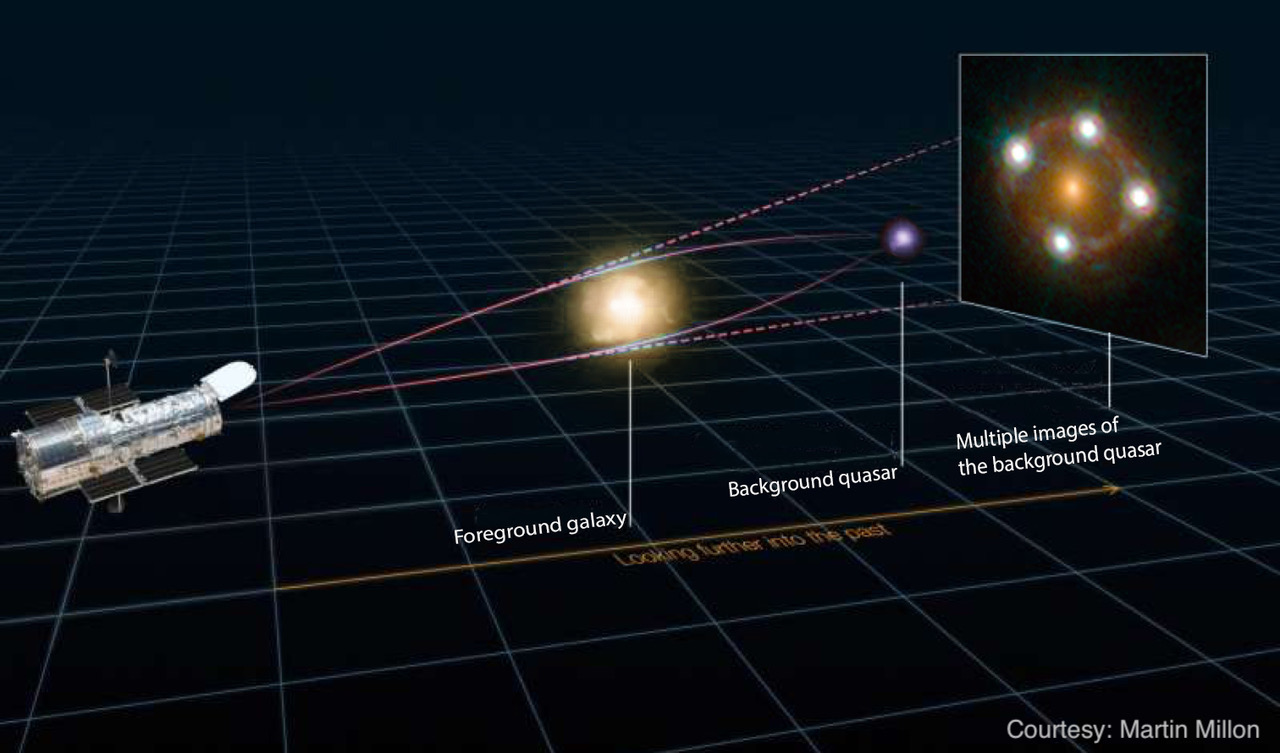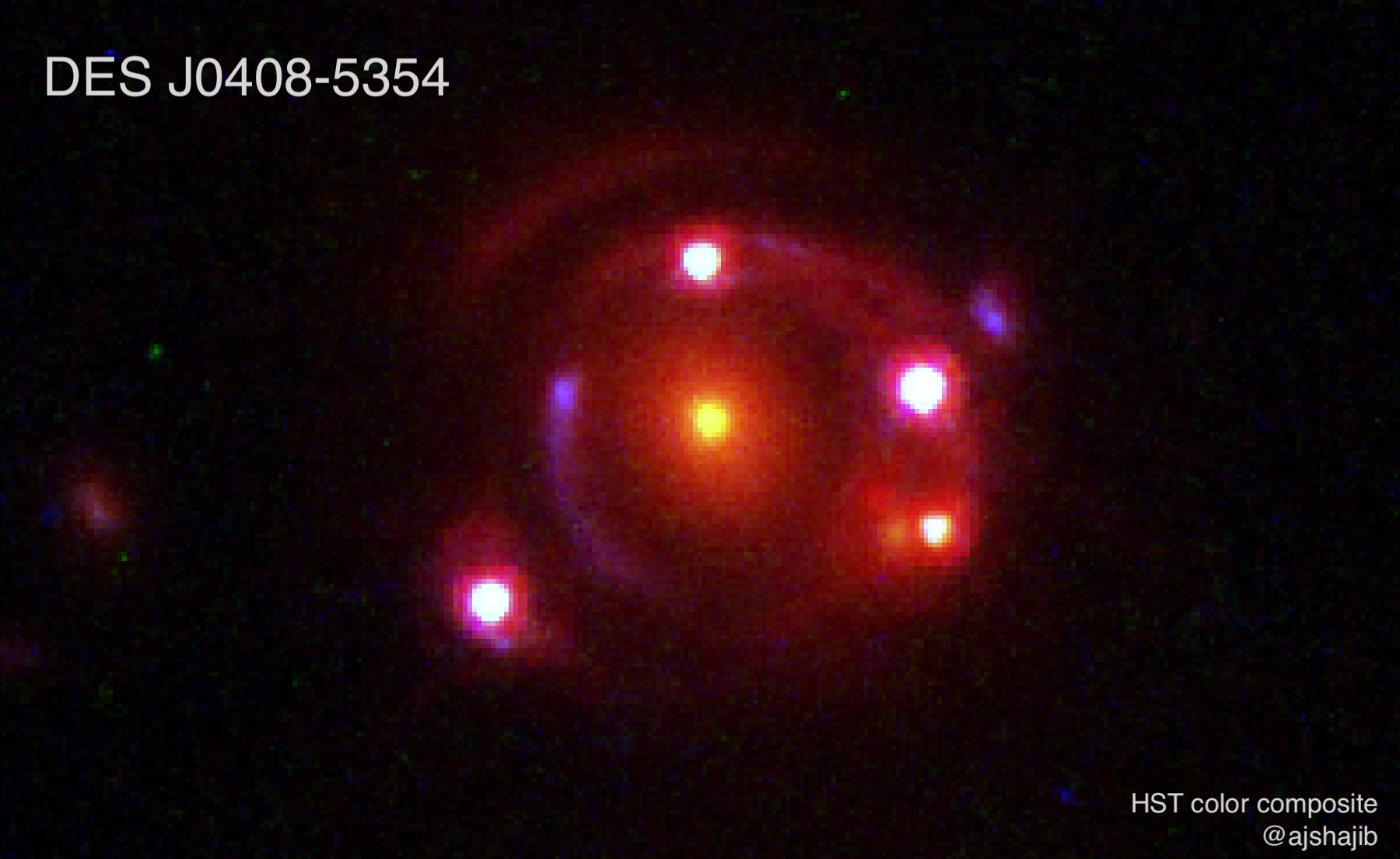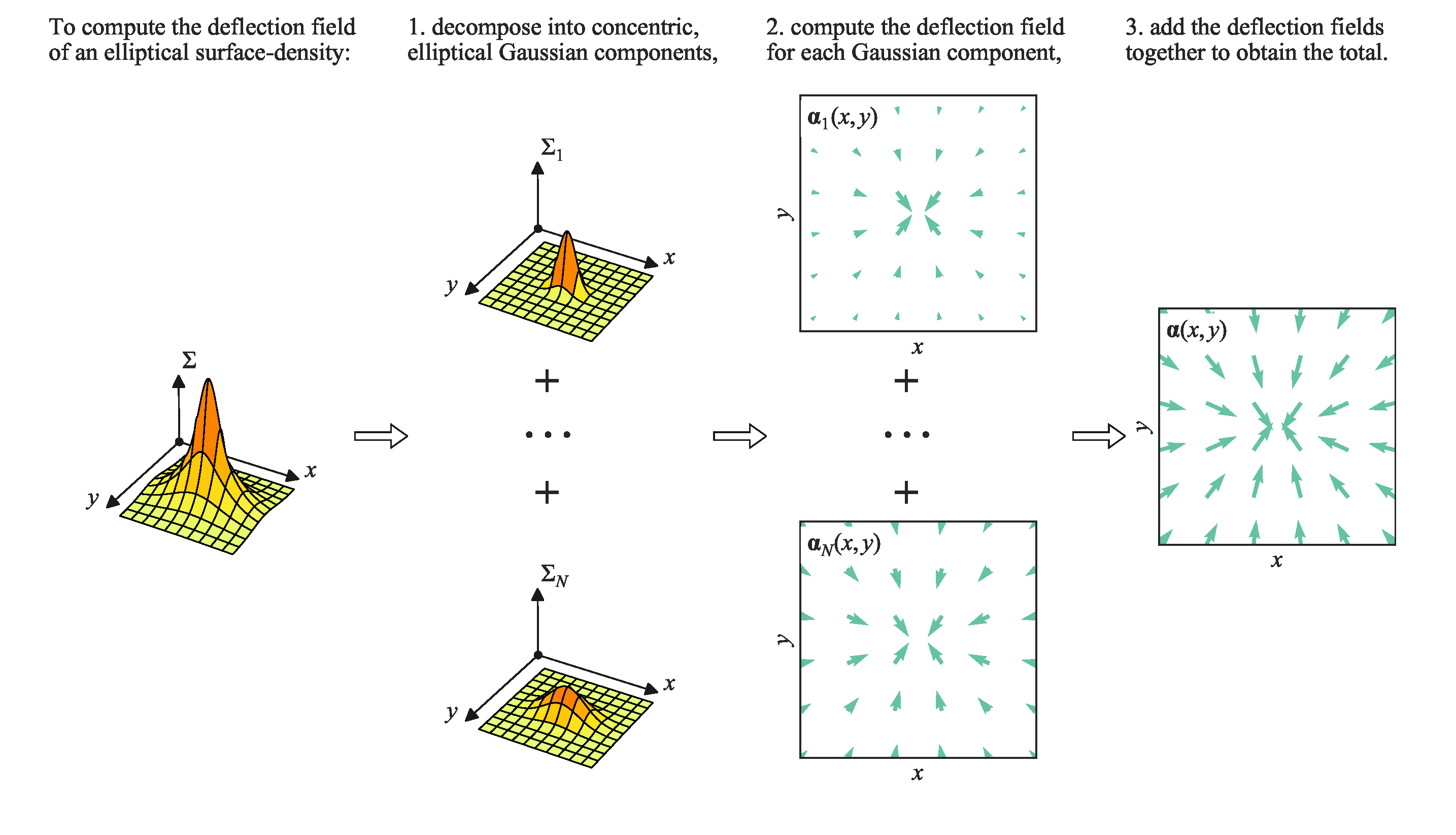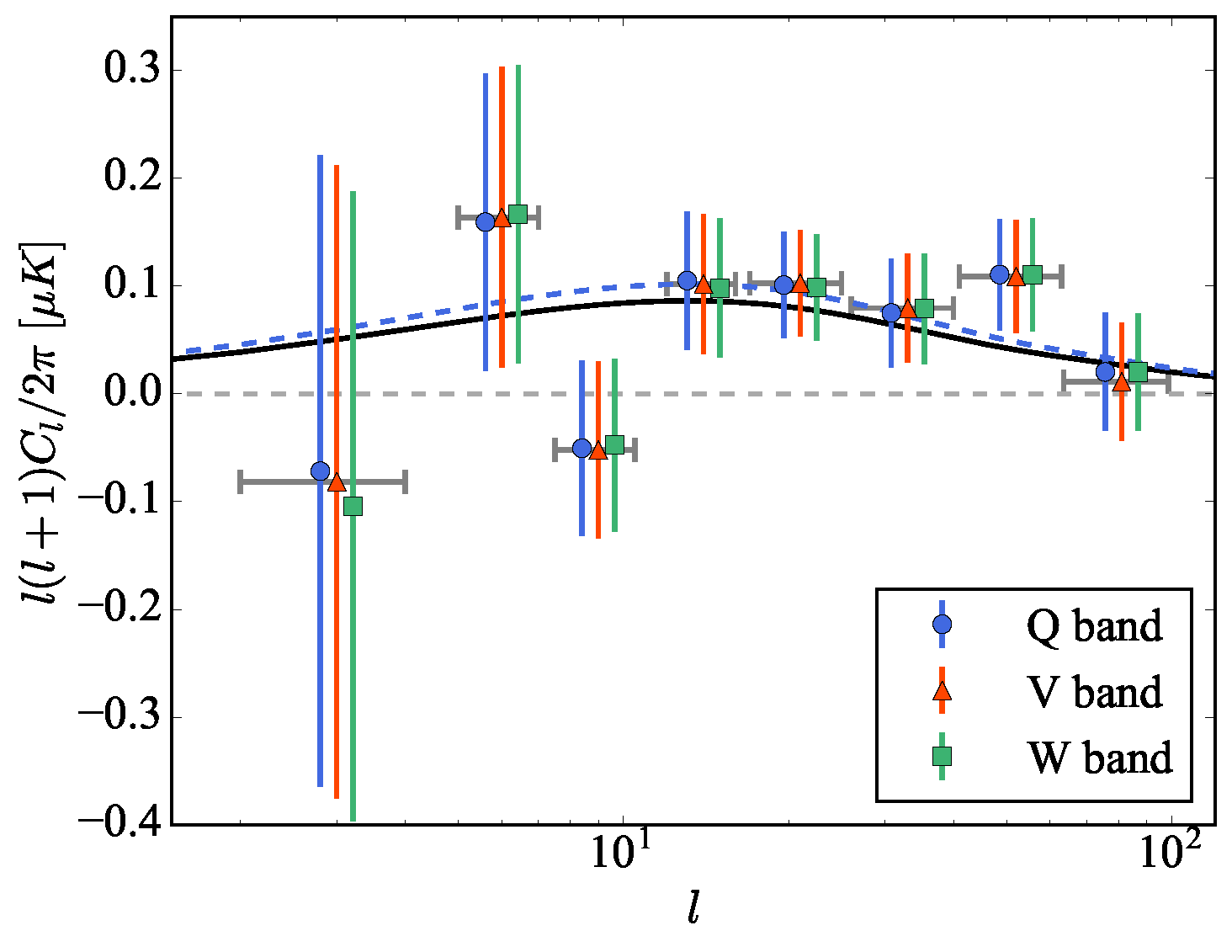I am a Postdoctoral Scholar in Astronomy and Astrophysics at the University of Chicago. My principle research interest is gravitational lensing and observational cosmology. I work with galaxy-galaxy strong lenses and strongly lensed quasar systems to study properties of elliptical galaxies at the intermediate redshift ($$z\sim0.5$$) and to measure cosmological parameters such as the Hubble constant. I am a co-PI of the STRIDES collaboration that aims to discover strongly lensed quasars from the Dark Energy Survey and other large-area sky-surveys.

I was born and raised in Bangladesh. I moved to Japan after graduating high school and received my B.S. degree in physics from the University of Tokyo in 2014. I completed my Phd in Astronomy and Astrophysics at the University of California, Los Angeles in 2020.

### Research#### Time-delay cosmography

Strong gravitational lensing is the phenomenon of light from a background object being deflected by the gravitational potential of a massive foreground object to form multiple images of the background object (left picture). If the background object is a quasar, then the delay between the arrival times of photon for different images can be measured using the intrinsic quasar variablity. This time-delay measurement can be used to estimate the so-called "time-delay distance". The time-delay distance constraints cosmological parameters, specially the Hubble constant $$H_0$$.#### The most precise single-lens $$H_0$$ measurement to date

I led a team from the STRIDES collaboration to measure $$H_0$$ from a recently discovered lens system (Shajib et al. 2019). This lens system, DES J0408$$-$$5354, is a very rare double-source system (left picture). Exploiting the richness of information in this system, we measured the Hubble constant $$H_0=74.2^{+2.7}_{-3.0}$$ km/s/Mpc with the highest precision (3.9%) from a single lens system to date.#### Efficient computation for elliptical lensing: solution to a 30-year-old problem

The 2D integral to compute the deflection angle for an elliptical mass profile is non-analytical in the general case, which lacked an efficient solution for more than three decades. I proposed an efficient method that solves this problem (Shajib 2019). My proposed framework self-consistently unifies the lensing and kinematic analyses of a general elliptical mass distribution.#### Integrated Sachs-Wolfe effect

In a dark-energy-dominated universe, the large-scale potential well decays with time. CMB photons gain a little amount of energy after crossing such a decaying potential well, which is the integrated Sachs-Wolfe (ISW) effect. I analyzed WISE and WMAP data to detect the ISW effect signal at $$3.4\sigma$$ S/N (left picture, Shajib & Wright 2016). This was the highest-significance detection of the ISW effect from a single dataset at the time of publication (2016).

### Publications

Total 21 papers: 7 first-author papers, 1 second author-papers, and 13 nth-author papers.

First author publications:
1. Shajib, A. J., et al. Massive elliptical galaxies at $$z\sim0.2$$ are well described by stars and a Navarro--Frenk--White dark matter halo. arXiv:2008.11724, 2020.
2. Shajib, A. J., et al. High-resolution imaging follow-up of doubly imaged quasars. arXiv:2011.01971, 2020
3. Shajib, A. J., et al. STRIDES: A 3.9 per cent measurement of the Hubble constant from the strong lens system DES J0408$$-$$5354. arXiv:1910.06306, 2019.
4. Shajib, A. J. Unified lensing and kinematic analysis for any elliptical mass profile. MNRAS, stz1796, 2019.
5. Shajib, A. J., et al. Is every strong lens model unhappy in its own way? Uniform modelling of a sample of 13 quadruply+ imaged quasars. MNRAS, 483, 5649-5671, 2019.
6. Shajib, A. J., Treu, T., and Agnello, A. Improving time-delay cosmography with spatially resolved kinematics. MNRAS, 473, 210-226, 2018.
7. Shajib, A. J. and Wright, E. L. Measurement of the integrated Sachs-Wolfe effect using the AllWISE data release. ApJ, 827:116, 2016.
Visit ADS for my complete publication list.

Find my CV here.